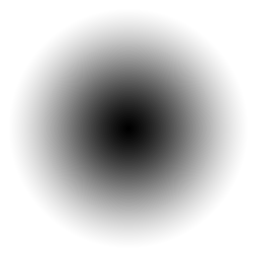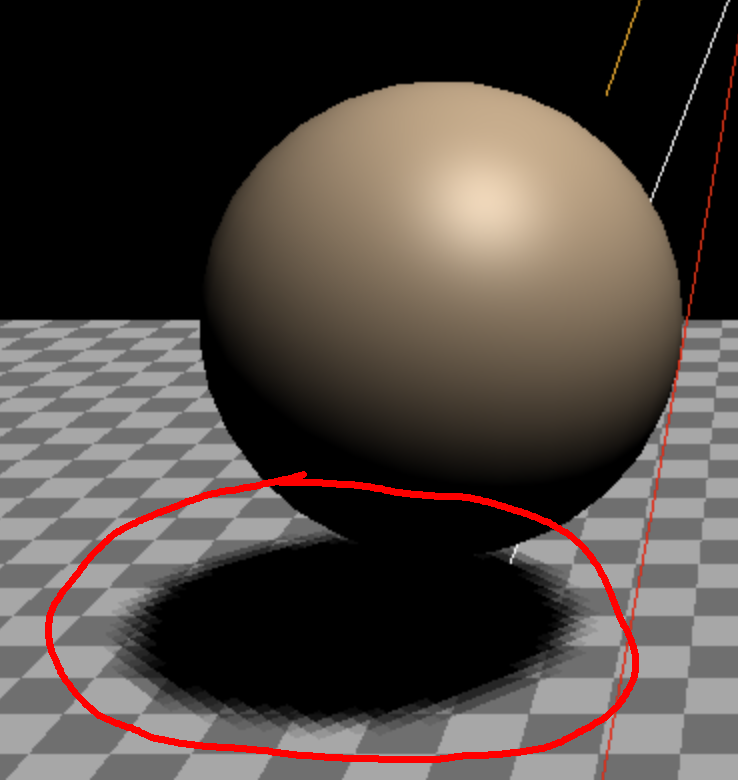# 阴影```const scene = new THREE.Scene();
+scene.background = new THREE.Color("white");
```

```+const loader = new THREE.TextureLoader();

{
const planeSize = 40;

texture.wrapS = THREE.RepeatWrapping;
texture.wrapT = THREE.RepeatWrapping;
texture.magFilter = THREE.NearestFilter;
const repeats = planeSize / 2;
texture.repeat.set(repeats, repeats);

const planeGeo = new THREE.PlaneGeometry(planeSize, planeSize);
const planeMat = new THREE.MeshBasicMaterial({
map: texture,
side: THREE.DoubleSide,
});
+  planeMat.color.setRGB(1.5, 1.5, 1.5);
const mesh = new THREE.Mesh(planeGeo, planeMat);
mesh.rotation.x = Math.PI * -.5;
}
```

```const shadowTexture = loader.load("resources/images/roundshadow.png");
```

and make an array to remember each sphere and associated objects. 并且创建一个数组来存放每个球体和它相关的对象

```const sphereShadowBases = [];
```

```const sphereRadius = 1;
const sphereWidthDivisions = 32;
const sphereHeightDivisions = 16;
const sphereGeo = new THREE.SphereGeometry(
sphereWidthDivisions,
sphereHeightDivisions
);
```

```const planeSize = 1;
const shadowGeo = new THREE.PlaneGeometry(planeSize, planeSize);
```

```const numSpheres = 15;
for (let i = 0; i < numSpheres; ++i) {
// make a base for the shadow and the sphere
// so they move together.
const base = new THREE.Object3D();

// add the shadow to the base
// note: we make a new material for each sphere
// so we can set that sphere's material transparency
// separately.
const shadowMat = new THREE.MeshBasicMaterial({
transparent: true, // so we can see the ground
depthWrite: false, // so we don't have to sort
});
shadowMesh.position.y = 0.001; // so we're above the ground slightly
shadowMesh.rotation.x = Math.PI * -0.5;

// add the sphere to the base
const u = i / numSpheres; // goes from 0 to 1 as we iterate the spheres.
const sphereMat = new THREE.MeshPhongMaterial();
sphereMat.color.setHSL(u, 1, 0.75);
const sphereMesh = new THREE.Mesh(sphereGeo, sphereMat);
sphereMesh.position.set(0, sphereRadius + 2, 0);

// remember all 3 plus the y position
base,
sphereMesh,
y: sphereMesh.position.y,
});
}
```

```{
const skyColor = 0xb1e1ff; // light blue
const groundColor = 0xb97a20; // brownish orange
const intensity = 2;
const light = new THREE.HemisphereLight(skyColor, groundColor, intensity);
}
```

The other is a `DirectionalLight` so the spheres get some definition 另一个是 `DirectionalLight` ，这将让球体看起来有些视觉的区别

```{
const color = 0xffffff;
const intensity = 1;
const light = new THREE.DirectionalLight(color, intensity);
light.position.set(0, 10, 5);
light.target.position.set(-5, 0, 0);
}
```

```function render(time) {
time *= 0.001;  // convert to seconds

...

const {base, sphereMesh, shadowMesh, y} = sphereShadowBase;

// u is a value that goes from 0 to 1 as we iterate the spheres
const u = ndx / sphereShadowBases.length;

// compute a position for the base. This will move
// both the sphere and its shadow
const speed = time * .2;
const angle = speed + u * Math.PI * 2 * (ndx % 1 ? 1 : -1);
const radius = Math.sin(speed - ndx) * 10;
base.position.set(Math.cos(angle) * radius, 0, Math.sin(angle) * radius);

// yOff is a value that goes from 0 to 1
const yOff = Math.abs(Math.sin(time * 2 + ndx));
// move the sphere up and down
sphereMesh.position.y = y + THREE.MathUtils.lerp(-2, 2, yOff);
// fade the shadow as the sphere goes up
shadowMesh.material.opacity = THREE.MathUtils.lerp(1, .25, yOff);
});

...
```

```const renderer = new THREE.WebGLRenderer({ canvas });
```

```const light = new THREE.DirectionalLight(color, intensity);
```

```const mesh = new THREE.Mesh(planeGeo, planeMat);
```

```const mesh = new THREE.Mesh(cubeGeo, cubeMat);

...

const mesh = new THREE.Mesh(sphereGeo, sphereMat);
```

```const cameraHelper = new THREE.CameraHelper(light.shadow.camera);
```

```class DimensionGUIHelper {
constructor(obj, minProp, maxProp) {
this.obj = obj;
this.minProp = minProp;
this.maxProp = maxProp;
}
get value() {
return this.obj[this.maxProp] * 2;
}
set value(v) {
this.obj[this.maxProp] = v / 2;
this.obj[this.minProp] = v / -2;
}
}
```

```const gui = new GUI();
gui.addColor(new ColorGUIHelper(light, 'color'), 'value').name('color');
gui.add(light, 'intensity', 0, 2, 0.01);
+{
+  folder.open();
+  folder.add(new DimensionGUIHelper(light.shadow.camera, 'left', 'right'), 'value', 1, 100)
+    .name('width')
+    .onChange(updateCamera);
+  folder.add(new DimensionGUIHelper(light.shadow.camera, 'bottom', 'top'), 'value', 1, 100)
+    .name('height')
+    .onChange(updateCamera);
+  const minMaxGUIHelper = new MinMaxGUIHelper(light.shadow.camera, 'near', 'far', 0.1);
+  folder.add(minMaxGUIHelper, 'min', 0.1, 50, 0.1).name('near').onChange(updateCamera);
+  folder.add(minMaxGUIHelper, 'max', 0.1, 50, 0.1).name('far').onChange(updateCamera);
+}
```

```function updateCamera() {
// update the light target's matrixWorld because it's needed by the helper
light.target.updateMatrixWorld();
helper.update();
// update the light's shadow camera's projection matrix
// and now update the camera helper we're using to show the light's shadow camera
cameraHelper.update();
}
updateCamera();
```

`width``height`的值设置在 30 附近，我们将看到阴影是正常渲染的，因为这个区域都在阴影相机的投影范围内。```-const light = new THREE.DirectionalLight(color, intensity);
+const light = new THREE.SpotLight(color, intensity);
```

```{
const cubeSize = 30;
const cubeGeo = new THREE.BoxGeometry(cubeSize, cubeSize, cubeSize);
const cubeMat = new THREE.MeshPhongMaterial({
color: "#CCC",
side: THREE.BackSide,
});
const mesh = new THREE.Mesh(cubeGeo, cubeMat);
mesh.position.set(0, cubeSize / 2 - 0.1, 0);
}
```

```-const light = new THREE.SpotLight(color, intensity);
+const light = new THREE.PointLight(color, intensity);

....

// so we can easily see where the point light is
+const helper = new THREE.PointLightHelper(light);